# Web全景图的原理及实现

2,504 0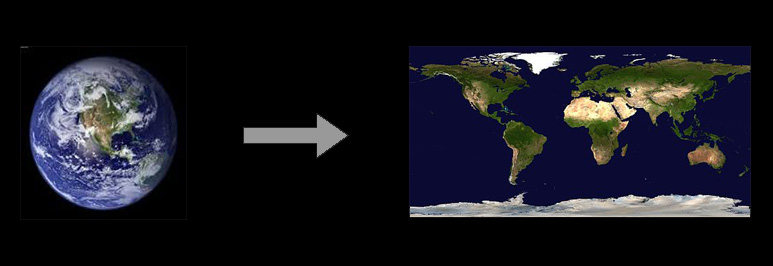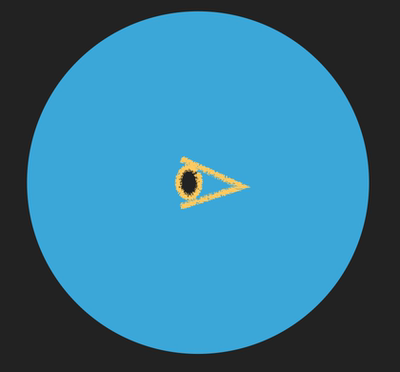``````//新建一个球体
var geometry = new THREE.SphereGeometry( 500, 60, 40 );
//沿x轴进行-1的scale，让球体的面朝内（因为我们将从球内进行观看）。
geometry.scale( - 1, 1, 1 );
//载入一张全景图生成threejs中可以使用的材质
var material = new THREE.MeshBasicMaterial( {
} );
//将几何体和材质进行结合。
mesh = new THREE.Mesh( geometry, material );``````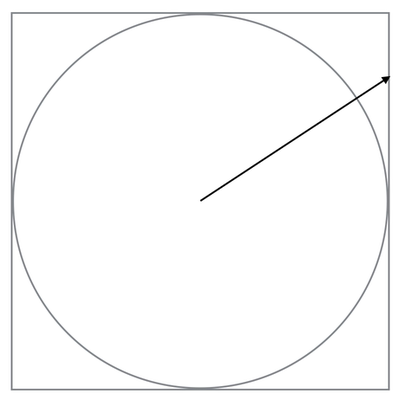``````r=1
0 < θ < π
-π/4 < ø < 7π/4``````

``````x= r sin θ cos ø
y= r sin θ sin ø
z= r cos θ``````

``1=Rsin θ cos ø``

``R= 1/(sin θ cos ø)``

``(1, tan ø, cot θ / cos ø)``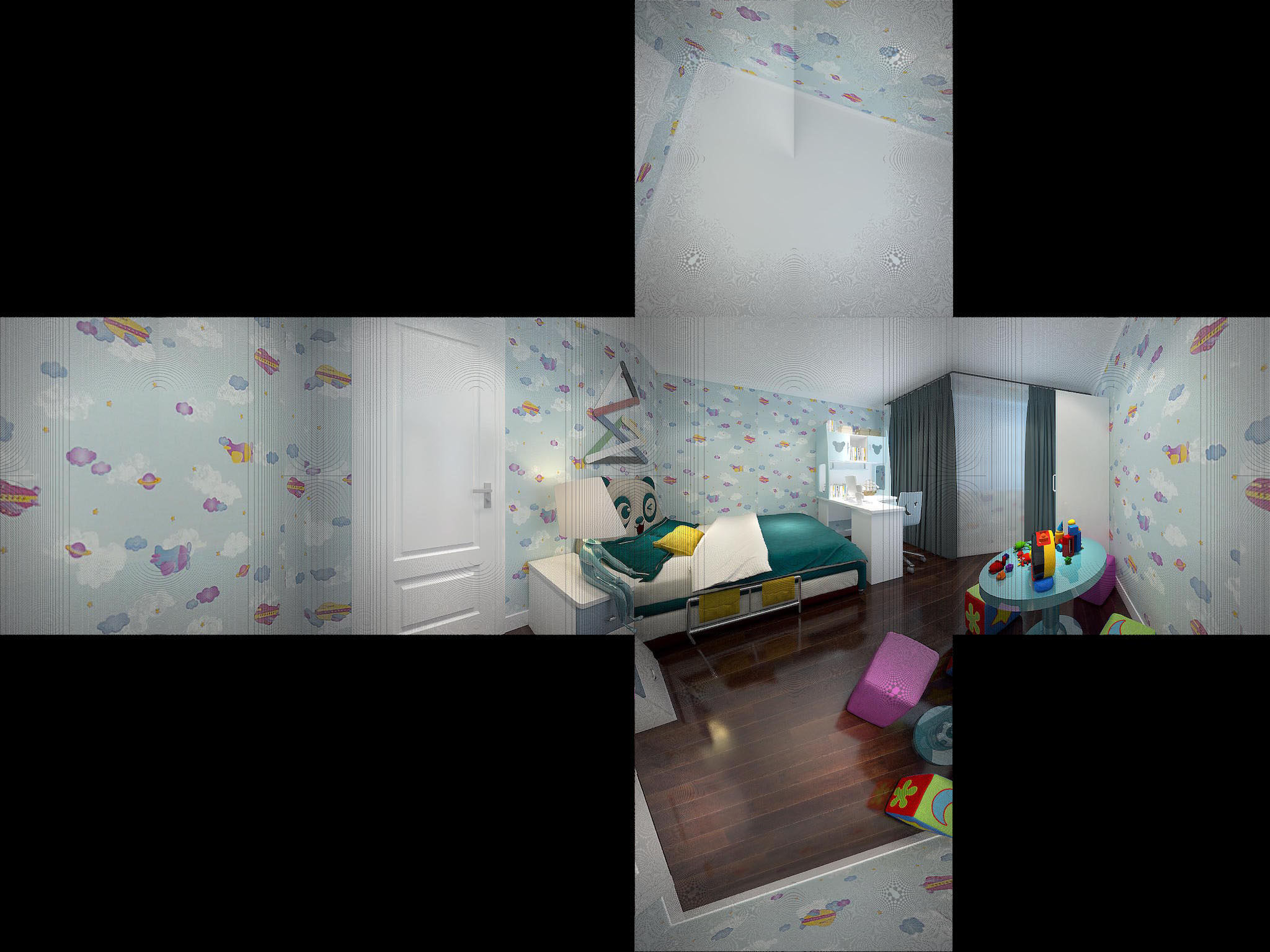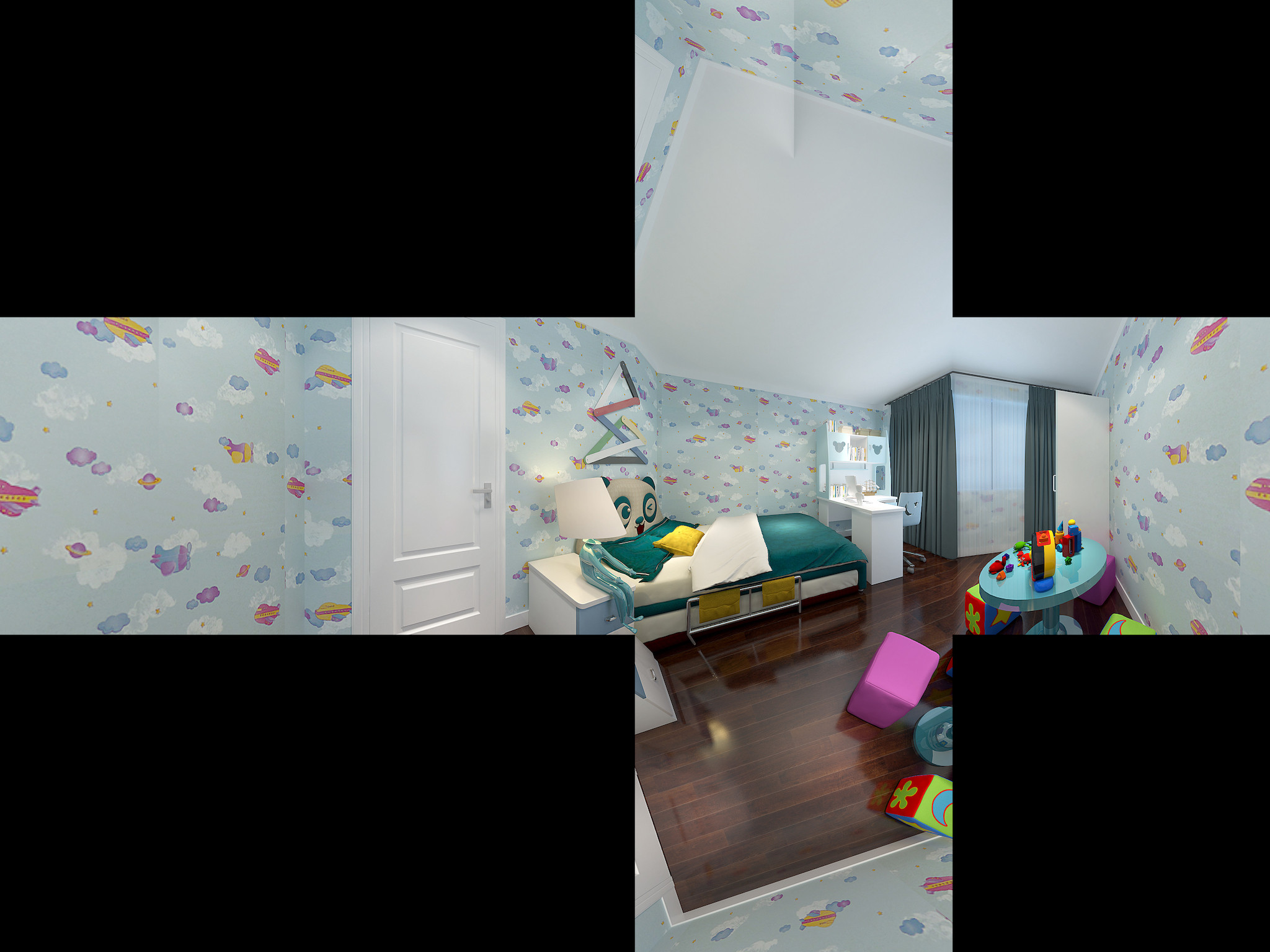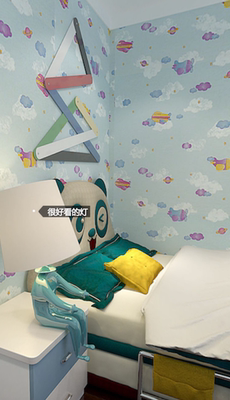``````//将鼠标点击事件中的位置信息，转换到位置中心
var mouse = new THREE.Vector2(
( ev.clientX / _this.wrapper.width() ) * 2 - 1,
-( ev.clientY / _this.wrapper.height() ) * 2 + 1
)``````

``````//创建一个Raycaster实例
var raycaster = new THREE.Raycaster()
//根据点击的位置，从镜头开始初始化一和镜头的屏幕垂直的直线
raycaster.setFromCamera(mouse, _this.camera)
//获得和直线相交的物体
var intersects = raycaster.intersectObjects(_this.scene.children)``````

``````//根据上一步中记录的位置生成一个向量
var vector  = new THREE.Vector3(pos.x, pos.y, pos.z)
//将这个向量映射到镜头的平面上
vector.project(camera)
//将位置信息还原成以左上角为原点的位置信息
var screenPos = {
left: Math.round((   vector.x + 1 ) * wrapper.width() / 2),
top: Math.round(( -vector.y + 1 ) * wrapper.height() / 2)
}``````